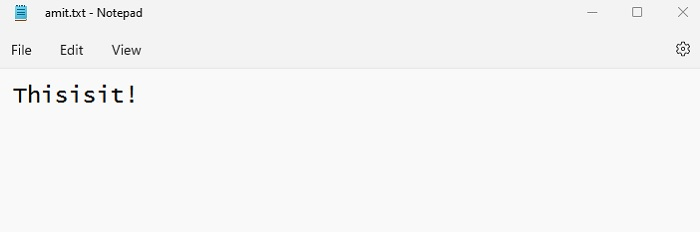# What does [::-1] do in Python?

Slicing in Python gets a sub-string from a string. The slicing range is set as parameters i.e. start, stop and step. For slicing, the 1st index is 0.

For negative indexing, to display the 1st element to last element in steps of 1 in reverse order, we use the [::-1]. The [::-1] reverses the order.

In a similar way, we can slice strings like this.

# slicing from index start to index stop-1
arr[start:stop]

# slicing from index start to the end
arr[start:]

# slicing from the beginning to index stop - 1
arr[:stop]

# slicing from the index start to index stop, by skipping step
arr[start:stop:step]

# slicing from 1st to last in steps of 1 in reverse order
arr[::-1]


Remember, negative number for step means "in reverse order". Let us now see examples −

## Reverse the order of a string in Python

Use the [::-1] to reverse the order of a string in Python −

### Example

myStr = 'Hello! How are you?'
print("String = ", myStr)

# Slice
print("Reverse order of the String = ", myStr[::-1])


### Output

String =  Hello! How are you?
Reverse order of the String =  ?uoy era woH !olleH


## Reverse the rows of a Pandas DataFrame

### Example

Use the [::-1] to reverse the rows of a dataframe in Python -

import pandas as pd

# Create a Dictionary
dct = {'Rank':[1,2,3,4,5], 'Points':[100,87, 80,70, 50]}

# Create a DataFrame from Dictionary elements using pandas.dataframe()
df = pd.DataFrame(dct)
print("DataFrame = \n",df)

# Reverse the DataFrame using [::-1]
print("\nReverse the DataFrame = \n",df[::-1])


### Output

DataFrame =
Rank  Points
0     1     100
1     2      87
2     3      80
3     4      70
4     5      50Reverse the DataFrame =
Rank  Points
4     5      50
3     4      70
2     3      80
1     2      87
0     1     100


## Display the contents of a text file in Reverse Order with Slicing

We will display the contents of a text file in reverse order. For that, let us first create a text file amit.txt with the following content -### Example

Let us now read the contents of the above file in reverse order -

# The file to be read
with open("amit.txt", "r") as myfile:

# Reversing the data by passing -1 for [start: end: step]
rev_data = my_data[::-1]

# Displaying the reversed data
print("Reversed data = ",rev_data)


### Output

Reversed data = !tisisihT